#### How to Find Outliers in Excel

In this article, we will learn how to find outliers in Excel.

## What are Outliers?

Outliers are the values in the data which are outside the scope of the general data values, meaning that they are very much higher than very much lower than the general data values.

## Why do we need to remove the Outliers?

Outliers spoil our data and representation. When we create a graph of complete data, if some values are extremely high and extremely low, the other values/range of the graph do not look good.

Let us create simple data. In this example, we can clearly see that some values are really outside, e.g., 200.

Let’s discuss the procedure to remove outliers. Let’s start by finding the first and third quartile:

1st quartile: =QUARTILE(A2:A14,1)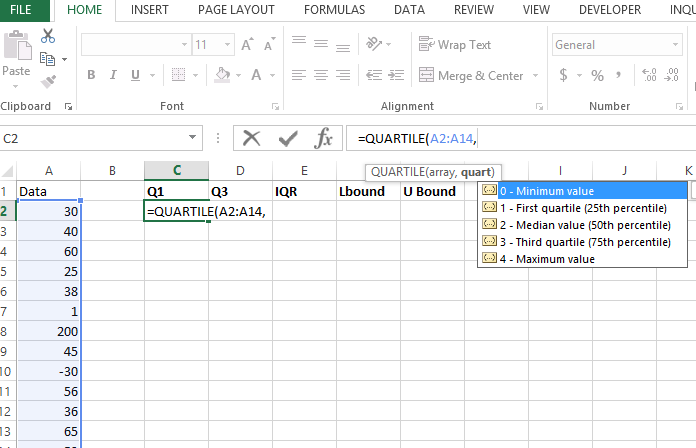3rd quartile: =QUARTILE(A2:A14,3)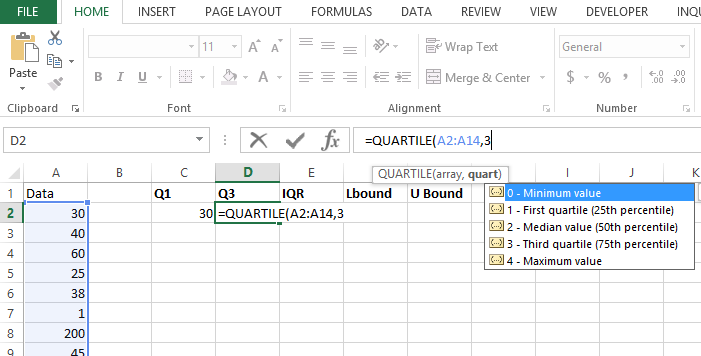## How to calculate interquartile range?

Let’s calculate the interquartile range (IQR). Because it is a range, you just need to subtract values. Q3-Q1.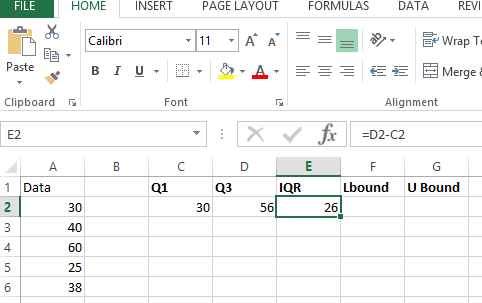Calculating IQR gives us the possibility to calculate the lower and upper bounds of data.

Let us calculate the lower bound and upper bound values:

Lower bound = lower of Q1 or Q3 – 1.5 * IQR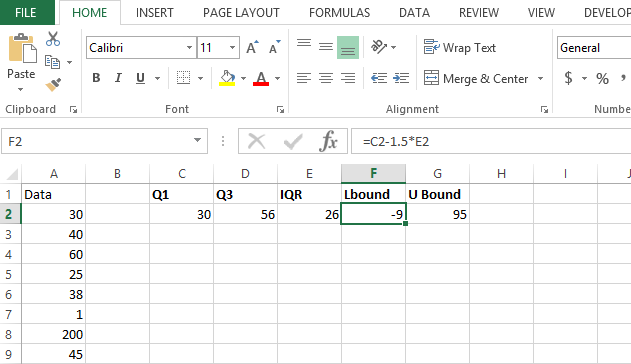Upper bound = max of Q1 or Q3 + 1.5 * IQR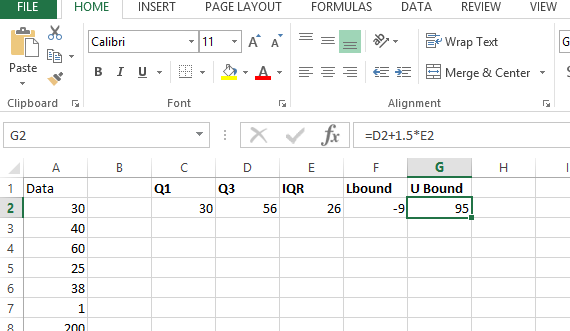Let us find the outlier by using the U bound and L bound: =OR(A2<\$F\$2,A2>\$G\$2)

Anything lower than the lower bound and higher than the upper bound is an outlier.

## Alternative methods

### Box Plot

A box plot, also known as a box-and-whisker plot, is a simple way to visualize the distribution of data and identify outliers. To create a box plot in Excel, you’ll need to use the “Q1”, “Q3”, and “MIN” and “MAX” functions.

### Standard deviation

Another method to find outliers is to use the standard deviation. If a value is more than a certain number of standard deviations away from the mean, it’s considered an outlier. To find outliers using this method, you’ll need to calculate the mean, standard deviation, and z-score for each value in your data set.

### Conditional formatting

A third option is to use conditional formatting to highlight outliers in your data set. To do this, you can use a formula to compare each value to a mean or median, and then format the cells that are above or below a certain threshold.

It’s important to note that the method you choose will depend on the type of data you’re working with and the results you’re trying to achieve. Each of these methods has its own strengths and limitations, so it’s important to understand each one and choose the method that best meets your needs.